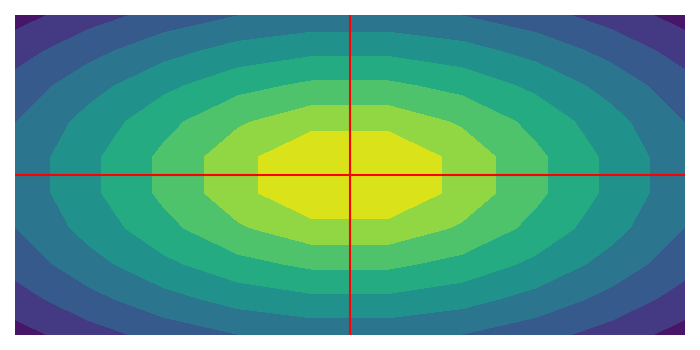# Draw axis lines or the origin for Matplotlib contour plot.

To draw axis lines or the origin for matplotlib contour plot, we can use contourf(), axhline() y=0 and axvline() x=0.

• Create data points for x, y, and z using numpy.

• To set the axes properties, we can use plt.axis('off') method.

• Use contourf() method with x, y, and z data points.

• Plot x=0 and y=0 lines with red color.

• To display the figure, use show() method.

## Example

import numpy as np
import matplotlib.pyplot as plt
plt.rcParams["figure.figsize"] = [7.00, 3.50]
plt.rcParams["figure.autolayout"] = True
x = np.linspace(-1.0, 1.0, 10)
x, y = np.meshgrid(x, x)
z = -np.hypot(x, y)
plt.axis('off')
plt.contourf(x, y, z, 10)
plt.axhline(0, color='red')
plt.axvline(0, color='red')
plt.show()

## Output